# Software

• Qaintum (2020 - …).
Digital quantum circuit simulation framework written in Julia (work in progress).

• Determinant quantum Monte Carlo (DQMC) (2015 - 2017).
C implementation and test files of the determinant quantum Monte Carlo (DQMC) method applied to Hubbard-type models, available at GitHub. This code has been used for the computations in the Science paper “Numerical evidence of fluctuating stripes in the normal state of high-Tc cuprate superconductors” [pdf].

• PyTeNet: A concise Python implementation of quantum tensor network algorithms (2018).
Python implementation of the matrix product state (MPS) framework, at GitHub. Features include the conversion of arbitrary “operator chains” into MPOs, real and imaginary time evolution based on the TDVP and built-in support for additive quantum numbers. See also the accompanying publication in the Journal of Open Source Software [doi].

• Quantum tensor networks, in particular matrix product states and operators (2013 - 2018).
C and Mathematica code available at GitHub. This implementation has been used for the simulations in the paper “Scrambling and thermalization in a diffusive quantum many-body system” [pdf].

•GuiTeNet: A graphical user interface for tensor networks (2018).
A graphical user interface for constructing arbitrary (quantum) tensor networks and specifying common operations like contractions or splitting, accessible at guitenet.org. JavaScript source code at GitHub.
See the corresponding preprint (arXiv:1808.00532) for a detailed description and a discussion of optimization strategies.

• Molecular dynamics simulations of classical anharmonic hard-point particle chains (2014).
C source code and Mathematica test files are available at GitHub.
This code has been used for the simulations in the papers “Current fluctuations for anharmonic chains in thermal equilibrium” [pdf] and “Equilibrium time-correlation functions for one-dimensional hard-point systems” [pdf].

• Nonlinear fluctuating hydrodynamics for anharmonic chains (2013 - 2014).
A Mathematica package and demonstration file for calculating the coupling constants is available at GitHub. See the paper by Herbert Spohn “Nonlinear fluctuating hydrodynamics for anharmonic chains” [pdf], as well as the papers “Equilibrium time-correlation functions for one-dimensional hard-point systems” [pdf] and “Dynamic correlators of Fermi-Pasta-Ulam chains and nonlinear fluctuating hydrodynamics” [pdf].

•FermiFab (2008 - 2020). Python, Matlab and Mathematica toolbox for many-particle quantum systems, available at GitHub (formerly at SourceForge). See the corresponding paper “The FermiFab toolbox for fermionic many-particle quantum systems” [pdf] for a detailed description.

• Matrix-valued quantum lattice Boltzmann method (summer 2013).
Lattice Boltzmann method (LBM) with quantum aspects.
The C source code and accompanying Mathematica file can be found here: [zip],
and the video shows a simulation from my paper [doi] (arXiv:1309.1656):

•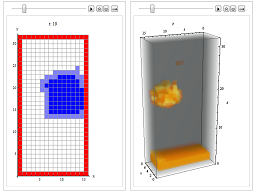Implementation of the classical lattice Boltzmann method (LBM) using the D2Q9 and D3Q19 models (2013 - 2014).
C implementation based on Nils Thürey’s PhD thesis “Physically based animation of free surface flows with the lattice Boltzmann method” [pdf]. C source code and demonstration Mathematica files are available at GitHub.

• Generating integral formulas from Feynman diagrams using Mathematica (summer 2013).
Mathematica code including demo file: [zip].
Based on the paper “Derivation of a Matrix-valued Boltzmann equation for the Hubbard model” (arXiv:1306.0934).
Automatically generate the integral expression corresponding to a given Feynman diagram. The implementation uses pattern matching, but is still rather preliminary and will be further extended.

•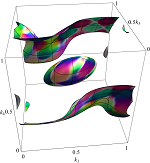Matrix-valued Boltzmann equation for the nonintegrable Hubbard chain (winter 2012/2013).
The C / Mathematica implementation of the simulations in the paper [pdf] is available at GitHub.
A challenging part was the numerical discretization of the collision manifold such that the analytic symmetries are preserved.

•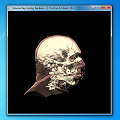Volume Ray-Casting Renderer with Transfer Functions (DirectX implementation, summer 2012).
Source code and binary files: [zip]. For demonstration purposes, not (yet) optimized for performance.
Based on the tutorial Volume Rendering 102: Transfer Functions by Kyle Hayward.

•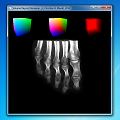Volume Ray-Casting Renderer (DirectX implementation, summer 2012).
Source code and binary files: [zip]. Note that the vertices of the virtual cube containing the volume voxel data do not use texture coordinates.
Inspired by the article Volume Rendering 101.
Minimum system requirements: DirectX 11 capable graphics card, and the “DirectX End-User Runtimes (June 2010)” (extract and run DXSetup.exe) on Windows 7.

• Simple Multigrid implementation using Matlab (spring 2012).
Matlab code available at GitHub. Simple, pedagogical implementation of the Multigrid method for solving Poisson-like equations. Reference: Ulrich Trottenberg, Cornelius W. Oosterlee, Anton Schuller. Multigrid, Academic Press (2001).

• Quantum Espresso UPF (Unified Pseudopotential Format) Parser and XML exporter, both Java and C# (spring 2012).
Source code and binary files of the Java version [zip], and the C# version [zip].
Produces a W3C-conforming XML file with tags for the variables (note that the original UPF format is not W3C-conforming).

• Wolfram Mathematica utility functions (spring 2012).
Some code snippets I’ve found useful.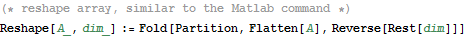Reshape array [cdf, html] Reshape an array, similar to the Matlab command Block diagonal matrix [cdf, html] Construct a block-diagonal matrix Tensor dot product [cdf, html] Generalized dot product for tensors Purge sparse array [cdf, html] “Purge” a sparse array by removing the redundant array rules
• Real-Time Radon Transform via the GPU Graphics Pipeline (fall 2010).
Implementation of the forward and inverse Radon transform in real-time on GPUs, using DirecX 11. A short manuscript [pdf] provides more details. The C++/shader source code, compiled binaries and project files (Visual Studio 2010) can be found here: [zip]. The corresponding paper “Comprehensive Analysis of High-Performance Computing Methods for Filtered Back-Projection” [pdf] is an extension to fan-beam geometry.Minimum system requirements: DirectX 10.0 capable graphics card, and the “DirectX End-User Runtimes (June 2010)” (extract and run DXSetup.exe) on Windows 7 or Vista (SP 2).

• Walking Biped Simulation v0.1 (Winter 2009 - January 2010).
C++ implementation using NVidia PhysX 2.8 and DirectX 10 (not perfect yet ;-)). Inspired by the TED talk “Torsten Reil builds better animations” (2003). The complete source code is available here: [zip].

• NSB Entropy Estimation (April - May 2009, updated September 2011).
A Mathematica and Matlab implementation of an algorithm for the entropy estimation from undersampled discrete data: [zip].
Reference: Ilya Nemenman, Fariel Shafee, William Bialek. Entropy and inference, revisited (arXiv:physics/0108025).

• C++ container classes (October 2005).
A platform independent collection of C++ container classes (similar to STL) with demo files: [zip], [tar]. Includes arrays, lists, sets, maps, hash sets, strings and a special array-like class which uses a red black tree to sort out multiple elements.

• Sharp3D (Summer 2004).
Developing a graphics engine “from scratch” using C#. Complete source code [zip], media files [zip]. Practical programming course at the Fakultät für Informatik, TUM. I’ve ported some DirectX vertex and pixel shaders.

• Enhancing Stenciled Shadow Volumes (August 2003).
Some technical details for storing vertex data in textures. I wrote this article as a high school student, so it’s pretty out of date.

•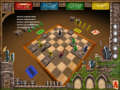Torres (May - August 2003). PC version of the board game of the year 2000. I’ve been part of the software development team at RadonLabs, Berlin.